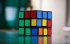×The Free 30-Day CAT RC Course

"It is designed to help you excel in the upcoming CAT 2023 exam"

-By Lavleen Kaur Kapoor. Over 1,73,000 Subscribers

No thanks >

OPEN

OPEN

## IBSAT, CAT, XAT, NMAT, GMAT

OPEN#### Get Personalized MBA Mentorship

Connect with my team on WhatsApp for unbiased MBA College Selection advice.

# QA-Cube (A Detailed Concept)CAT Registration Ends: 13th September 2023This year also in CAT 2021 which will be conducted in November 2021, QA (Quantitative Ability) will be a separate section.

Definition –

A solid with six congruent square faces. A regular hexahedron.

A cube is a region of space formed by six identical square faces joined along their edges. Three edges join at each corner to form a vertex. The cube can also be called a regular hexahedron. It is one of the five regular polyhedrons, which are also sometimes referred to as the Platonic solids.

Parts of a cube –

Face:

Also called facets or sides. A cube has six faces which are all squares, so each face has four equal sides and all four interior angles are right angles.

Edge:

A line segment formed where two edges meet. A cube has 12 edges. Because all faces are squares and congruent to each other, all 12 edges are the same length.

Vertex:

A point formed where three edges meet. A cube has 8 vertices.

Face Diagonals:

Face diagonals are line segments linking the opposite corners of a face. Each face has two, for a total of 12 in the cube.

Space Diagonals:

Space diagonals are line segments linking the opposite corners of a cube, cutting through its interior. A cube has 4 space diagonals.

Volume enclosed by a cube:

Definition –

The number of cubic units that will exactly fill a cube

How to find the volume of a cube?

Recall that a cube has all edges the same length. The volume of a cube is found by multiplying the length of any edge by itself twice. So if the length of an edge is 4, the volume is 4 x 4 x 4 = 64

Or as a formula; Volume = s3 where: S is the length of any edge of the cube.

Surface area of a cube:

Definition –

The number of square units that will exactly cover the surface of a cube

How to find the surface area of a cube

Recall that a cube has all edges the same length. This means that each of the cube's six faces is a square. The total surface area is therefore six times the area of one face.

Or as a formula; Surface area = 6s2 where: S is the length of any edge of the cube.